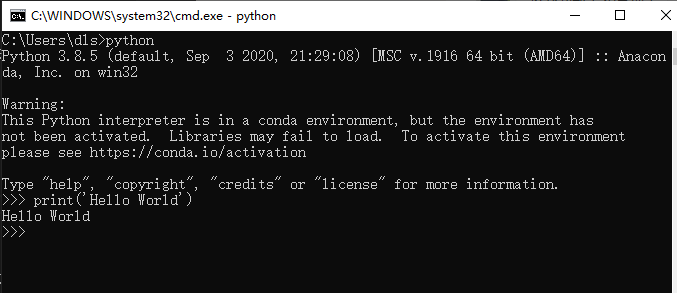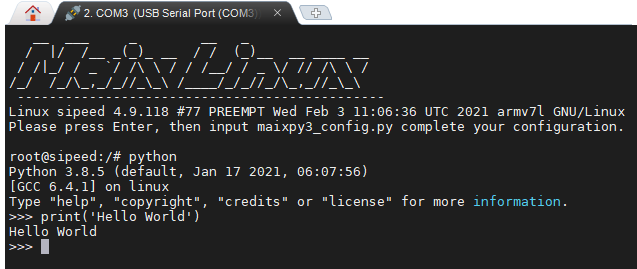## 学会循环执行代码

print('Hello World')print('Hello World')
print('Hello World')
print('Hello World')
print('Hello World')
print('Hello World')




while True:
print('Hello World')




while True:
try:
print('Hello World')
except Exception as e:
pass



## 循环代码中为什么需要异常机制

• 串口芯片损坏或线路断路、串口到芯片的通路损坏导致的串口没有数据输出。
• 串口线路数据不稳定、串口协议（波特率、停止位）等配置错误导致的数据乱码。

• 当网络连接失败后需要超时重连，传输数据通道闲置时需要定时检查心跳数据包。
• 当配置文件写入后通常会读出来确认真的写入了，也是为了防止出错，可能是存储介质出错，也可能是逻辑出错。
• 当用户向输入框填了错误数据，不用写怎么判断和处理，不合法的数据抛出异常就行。

• 检查当前的系统是否能联网
• 检查数据库的通路是否正常
• 检查指定的程序是否在运行

## 优化！优化！！优化！！！

def func():
i = 20**20000

import time
last = time.time()
func()
tmp = time.time() - last
print(tmp)


PS C:\Users\dls\Documents\GitHub\MaixPy3> & C:/Users/dls/anaconda3/python.exe c:/Users/dls/Documents/GitHub/MaixPy3/test.py
0.000997781753540039


root@sipeed:/# python3
Python 3.8.5 (default, Jan 17 2021, 06:07:56)
[GCC 6.4.1] on linux
>>> def func():
...     i = 20**20000
...
>>> import time
>>> last = time.time()
>>> func()
>>> tmp = time.time() - last
>>> print(tmp)
0.09001994132995605
>>>
>>>
>>> def func():
...     i = 20**20000
...
>>> import time
>>> last = time.time()
>>> func()
>>> print(time.time() - last)
1.480057954788208
>>>root@sipeed:/# cat test.py
def func():
i = 20**20000

import time
last = time.time()
func()
tmp = time.time() - last
print(tmp)

root@sipeed:/# python test.py
0.028677940368652344
root@sipeed:/#


### 讲一些经典案例


def xxxx_func():
import random
return random.randint(0, 1)

while True:
is_idle = True
if is_idle is True:
print('try start')
is_connected = xxxx_func()
if is_connected is True:
print('try connect')
is_send = xxxx_func()
if is_send is True:
print('try send')
is_exit = xxxx_func()
if is_exit is True:
print('operate successfully')


def xxxx_func():
return 1

# state value
is_idle, is_ready, is_connected, is_send, is_reply, is_exit = 0, 1, 2, 3, 4, 5
state = is_idle

while state != is_exit:

state = is_exit if xxxx_func() else is_send
continue

if state is is_send:
print('try send')
state = is_reply if xxxx_func() else is_connected
continue

if state is is_connected:
print('try connect')
state = is_send if xxxx_func() else is_ready
continue

state = is_connected if xxxx_func() else is_idle
continue

if state is is_idle:
print('try start')
continue


### 还有吗？


is_a, is_b, is_c = 0, 1, 2

state = is_a

def try_b():
global state
state = is_c

def try_a():
global state
state = is_b

func = [try_a, try_b]

while state != is_c:
func[state]()
# print(state)




class xxxx_fsm:

is_start, is_ready, is_connected, is_send, is_reply, is_exit = 0, 1, 2, 3, 4, 5

def xxxx_func(self):
return 1

def __init__(self):
self.state = __class__.is_start # state value

self.state = __class__.is_exit if self.xxxx_func() else __class__.is_send

def try_send(self):
self.state = __class__.is_reply if self.xxxx_func() else __class__.is_connected

def try_connect(self):
self.state = __class__.is_send if self.xxxx_func() else __class__.is_ready

self.state = __class__.is_connected if self.xxxx_func() else __class__.is_start

def try_start(self):

def event(self):
self.func[self.state]()

def check(self):
return self.state != __class__.is_exit

tmp = xxxx_fsm()

while tmp.check():

tmp.event()

# print(tmp.state)


### 说说异步的执行方式

import time, random

def check_http():
time.sleep(random.randint(0, 3))
return random.randint(0, 1)

def http_recv():
while True:
if check_http():
print('http_recv')
break

def check_key():
time.sleep(random.randint(0, 2))
return random.randint(0, 1)

def key_press():
while True:
if check_key():
print('key_press')
break

while True:
http_recv()
key_press()


import time, random

http_state, key_state = 0, 0

def http_recv():
global http_state
if http_state:
print('http_recv')

def key_press():
global key_state
if key_state:
print('key_press')

def check_state():
global key_state, http_state
time.sleep(random.randint(0, 1))
key_state, http_state = random.randint(0, 2), random.randint(0, 2)

while True:
check_state()
http_recv()
key_press()


import asyncio

await asyncio.sleep(tm)
print('%s over...' % name)

async def main(name):
import time
last = time.time()
await asyncio.gather(
)
print(name, time.time() - last)

loop = asyncio.get_event_loop()
tasks = [ main('l: '), main('r: ') ]



PS python.exe test.py
r: A over...
l: A over...
r: B over...
l: B over...
r: C over...
l: C over...
r:  0.3076450824737549
l:  0.3076450824737549



import asyncio, random

async def key_press():
await asyncio.sleep(0.1)
key_state = random.randint(0, 1)
if key_state:
return 'have key_press'

async def http_recv():
await asyncio.sleep(0.2)
http_state = random.randint(0, 1)
if http_state:
return 'have http_recv'

async def run():
import time
while True:
done, pending = await asyncio.wait(task_list, timeout=random.randint(0, 1) / 2)
await asyncio.sleep(0.2) # remove to run too fast.

loop = asyncio.get_event_loop()
loop.run_until_complete(run())


1615141673.93252 [None, None]
1615141674.134 [None, 'have http_recv']
1615141674.3350334 [None, None]
1615141674.7361133 ['have key_press', 'have http_recv']
1615141674.9365196 [None, None]
1615141675.1399093 ['have http_recv', None]


## 考虑一下封装模块给其他人使用吧？

def code():
print('this is code')


info = 'nihao'


>>> import mod
>>> mod
<module 'mod' from 'C:\\mod\\__init__.py'>
>>> mod.code()
this is code
>>> from mod import tmp
>>> tmp
<module 'mod.tmp' from 'C:\\mod\\tmp.py'>
>>> tmp.info
'nihao'
>>>


## 如何进行内存上的分析？

from memory_profiler import profile

@profile
def my_func():
a =  * (10 ** 6)
b =  * (2 * 10 ** 7)
del b
return a


Line #    Mem usage    Increment  Occurences   Line Contents
============================================================
3   38.816 MiB   38.816 MiB           1   @profile
4                                         def my_func():
5   46.492 MiB    7.676 MiB           1       a =  * (10 ** 6)
6  199.117 MiB  152.625 MiB           1       b =  * (2 * 10 ** 7)
7   46.629 MiB -152.488 MiB           1       del b
8   46.629 MiB    0.000 MiB           1       return a